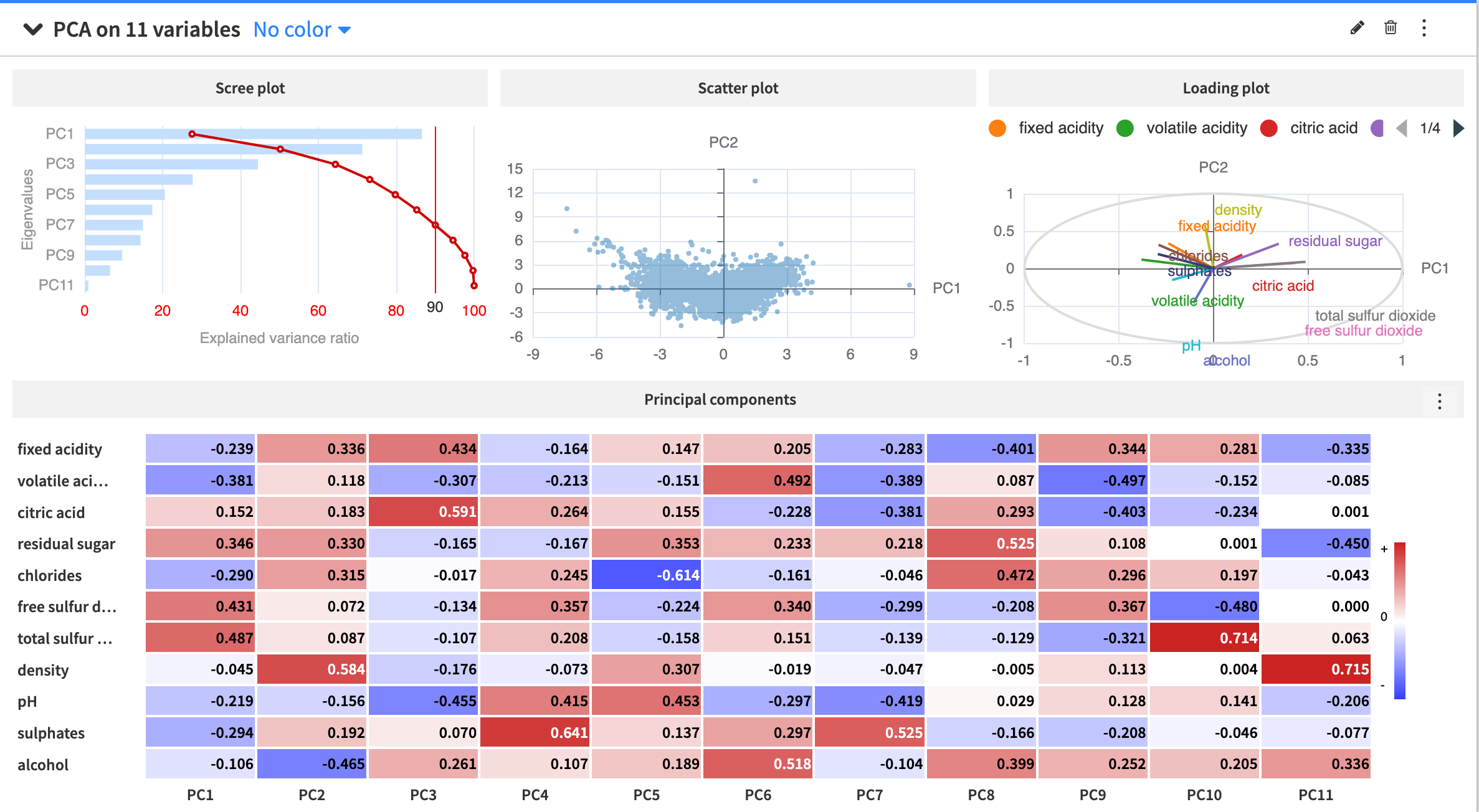# Tutorial | Analyze effects of dimensionality reduction with the PCA card¶

Note

This lesson is a continuation of the Interactive Visual Statistics hands-on tutorial.

When working with a dataset having many variables, we may be interested in analyzing the effects of using a reduced number of variables (or dimensions) of the data. For example, we may choose to explore the structure of the winequality dataset in two dimensions.

Dataiku enables you to analyze the effects of dimensionality reduction using a feature extraction method called Principal Component Analysis, or PCA.

## Perform PCA¶

Let’s use the Principal Component Analysis card to represent the winequality dataset in two dimensions.

• Click the New Card button from the Worksheet header and select Multivariate Analysis > Principal Component Analysis.

• Select the 11 numerical variables to add to the Variables column.

• Click Create Card to create the card.The scree plot in the PCA card shows that using only the first two principal components retains about 50.2% of the variance in the dataset. To retain a variance of at least 90% (the red vertical line), you must use a minimum of 7 principal components to represent the data.

The scatter plot shows the data projected onto the first two principal components.

The loading plot shows how strongly each of the 11 numerical variables influences the first two principal components. Vectors forming a small angle, such as volatile acidity and fixed acidity, are likely to be positively correlated. Vectors meeting in an orthogonal angle (or nearly orthogonal angle), such as density and total sulfur dioxide, are not likely to be correlated or have very little correlation. When two vectors form a large angle, such as residual sugar and pH, they are likely to be negatively correlated.

Lastly, the principal components heatmap shows a matrix of the principal component loading vectors. For instance, the first column of the matrix corresponds to the loading vector of PC1, or a vector of the coefficients used in the linear transformation of the data set to the first principal component dimension.

Note